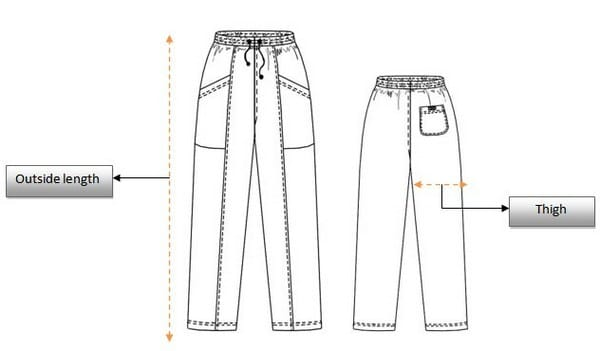# Fabric Consumption and Costing for 1 Dozen Trousers

Introduction:
Fabric consumption for making a trouser is an important task for garments merchandiser. When a buying house get an order for trouser then merchandising manager distribute order among other merchandisers. Then consumption is the first and basic work for responsible merchandiser.Figure: Trouser sketch

Fabric Consumption Calculation for 1 Dozen Trousers:
Equation for consumption of 1 piece trouser:

Fabric Consumption for trouser = (L + Allowance) x (W+ Allowance) x 4

Here,
L= Length of the out seam measurement including waist band = 45”,
Bottom hem seam allowance = 2”
Waist band seam allowance = 1”
Sewing allowance = 1”

W = Thigh width = 15”
Inseam allowance =1”
Out seam allowance = 1”

Now,
Fabric Consumption for trouser = (45+4) x (15+2) x 4
= 49 x 17 x 4 sq. inch
= 3332 sq. inch

Fabric consumption for pocketing:
It is also required to calculate pocketing and lining fabric requirement. As per design, two front pockets are considered. Calculation is as follows:

Fabric consumption for pocketing: (L+ 1) X (W+1) X Number of pockets

Here,
L = length of the pocket = 10”
W= Width of the pocket = 6”
Number of pockets = 2

Now,
Fabric consumption for pocketing = (10+ 1) X (6+1) X 2
= 11 x 7 x 2 sq. inch
= 154 sq. inch

Total fabric consumption for 1 piece trouser = 3332 + 154 sq. inch
= 3486 sq. inch

If we consider the width of fabric = 60 inch
Then marker width = 58, therefore

Fabric required for 1 piece trouser = 3486/58 inch
= 60.1 inch
= 60.1/36 yds
= 1.67 yds

If the wastage 9%,
Total consumption with waste percentage
= 1.67 + 9% yds
= 1.76 yds

1.76 yds fabric need for 1 piece trouser.

So, 1 Dozen trousers need = 12 x 1.76 = 21.12 yds fabric

Costing Calculation of 1 Dozen Trouser:

If,
Cost of main fabric = (21.12 x 2)\$ [\$2/yd] = 42.24\$
Cost of accessories/doz …..…………………… = \$7.00
Washing cost/doz ………………………………. = \$4.50
C. M. ………………………….……………………… = \$18.00
…………………………………………………………….……………………
Total cost                                                           = 71.74\$

1 Dozen trouser cost is 71.74\$.
Actual costing depends on accessories, washing and cost of making. And its depend on buyer requirements.